# HSSlive: Plus One & Plus Two Notes & Solutions for Kerala State Board

## AP Board Class 9 Maths Chapter 2 Polynomials and Factorisation Ex 2.5 Textbook Solutions PDF: Download Andhra Pradesh Board STD 9th Maths Chapter 2 Polynomials and Factorisation Ex 2.5 Book AnswersAP Board Class 9 Maths Chapter 2 Polynomials and Factorisation Ex 2.5 Textbook Solutions PDF: Download Andhra Pradesh Board STD 9th Maths Chapter 2 Polynomials and Factorisation Ex 2.5 Book Answers

## Andhra Pradesh State Board Class 9th Maths Chapter 2 Polynomials and Factorisation Ex 2.5 Books Solutions

 Board AP Board Materials Textbook Solutions/Guide Format DOC/PDF Class 9th Subject Maths Chapters Maths Chapter 2 Polynomials and Factorisation Ex 2.5 Provider Hsslive

2. Click on the Andhra Pradesh Board Class 9th Maths Chapter 2 Polynomials and Factorisation Ex 2.5 Answers.
3. Look for your Andhra Pradesh Board STD 9th Maths Chapter 2 Polynomials and Factorisation Ex 2.5 Textbooks PDF.
4. Now download or read the Andhra Pradesh Board Class 9th Maths Chapter 2 Polynomials and Factorisation Ex 2.5 Textbook Solutions for PDF Free.

## AP Board Class 9th Maths Chapter 2 Polynomials and Factorisation Ex 2.5 Textbooks Solutions with Answer PDF Download

Find below the list of all AP Board Class 9th Maths Chapter 2 Polynomials and Factorisation Ex 2.5 Textbook Solutions for PDF’s for you to download and prepare for the upcoming exams:

## AP State Syllabus 9th Class Maths Solutions 2nd Lesson Polynomials and Factorisation Exercise 2.5

Question 1.
Use suitable identities to find the following products.
i) (x + 5) (x + 2)
Solution:
(x + 5) (x + 2)
= x2 + (5 + 2)x + 5 x 2
[ ∵ (x + a) (x + b) = x2 + (a + b) x + ab]
= x2 + 7x + 10

ii) (x – 5) (x – 5)
Solution:
(x – 5) (x – 5)
= (x – 5)2 = x2 – 2(x) (5) + 52
[ ∵(x – y)2 = x2 – 2xy + y2]
= x2 – 10x + 25

iii) (3x + 2) (3x – 2)
Solution:
(3x + 2) (3x – 2) = (3x)2 – (2)2
[∵ (x + y) (x – y) =x– y2]
= 9x2 – 4

iv) (𝑥2+1𝑥2)(𝑥2−1𝑥2)
Solution: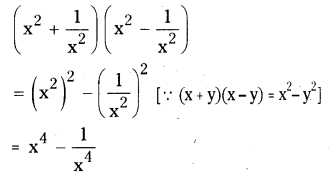v) (1 + x) (1 + x)
Solution:
(1 + x) (1 + x)
= (1 + x)2 = 12 + 2 (1) (x) + x2
[∵(x + y)2 = x2 + 2xy + y2]
= 1 + 2x + x2

Question 2.
Evaluate the following products with¬out actual multiplication.
i) 101 x 99
Solution:
101 x 99
= (100 + 1) (100 – 1)
= 1002 – 12
= 10000 – 1
= 9999

ii) 999 x 999
Solution:
999 x 999
= 9992
= (1000 – 1)2
= 10002 – 2 x (1000) x 1 + 12
= 1000000-2000 + 1
= 998001

iii) 5012×4912
Solution: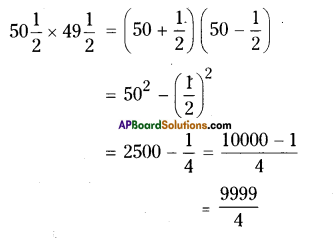iv) 501 x 501
Solution:
501 x 501
= (500 + 1) (500 + 1)
= (500 + 1)2
= 5002 + 2 x (500) x 1 + 12
= 250000 + 1000 + 1 = 251001

v) 30.5 x 29.5 = (30 + 0.5) (30 – 0.5)
= 302 – (0.5)2
= 900 – 0.25
= 899.75

Question 3.
Factorise the following using appro-priate identities.
i) 16x2 + 24xy + 9y2
Solution:
16x2 + 24xy + 9y2
= (4x)2 + 2 (4x) (3y) + (3y)2
= (4x + 3y)2 = (4x + 3y) (4x + 3y)
[ ∵ (x + y)2 = x2 + 2xy + y2]

ii) 4y2 – 4y + 1
Solution:
4y2 – 4y + 1
= (2y)2 – 2 (2y) (1) + (1)2
[ ∵ (x -y)2 = x2 – 2xy + y2]
= (2y -1)2 = (2y – 1) (2y-1)

iii) 4𝑥2−𝑦225
Solution: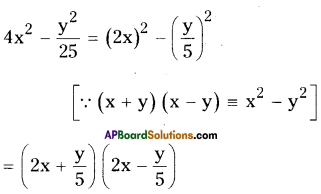iv) 18a2 – 50
Solution:
18a2 – 50 = 2 (9a2 – 25)
= 2[(3a)2 – (5)2]
[ ∵ x2 – y2 = (x + y) (x – y)]
= 2 (3a + 5) (3a – 5)

v) x2 + 5x + 6
Solution:
x2 + 5x + 6 = x2 + (3 + 2) x + 3 x 2
[ ∵ (x + a) (x + b) = x2 + (a + b) x + a . b]
= (x + 3) (x + 2)

vi) 3p2 – 24p + 36
Solution:
3p2 – 24p + 36
= 3[p2 – 8p + 12]
= 3[p2 + (- 6 – 2)p + (- 6) (- 2)]
[ ∵ (x + a) (x + b) = x2 + (a + b) x + ab]
= 3 (p – 6) (p – 2)

Question 4.
Expand each of the following, using suitable identities.
i) (x + 2y + 4z)2
(x + 2y + 4z)2 = (x)2 + (2y)2 + (4z)2 + 2(x) (2y) + 2 (2y) (4z) + 2 (4z) (x)
[ ∵ (x + y + z)2 = x2 + y2 + z2 + 2xy + 2yz + 2zx]
= x2 + 4y2 + 16z2 + 4xy + 16yz + 8zx

ii) (2a – 3b)3
Solution:
(2a – 3b)3 = (2a)3 – 3 (2a)2 (3b) + 3 (2a) (3b)2 – (3b)3
[ ∵ (a – b)3 = a3 – 3a2b + 3ab2 – b3]
= 8a3 – 3(4a2) (3b) + 3 (2a) (9b2) – 27b3
= 8a3 – 36a2b + 54ab2-27b3
(or)
∵ (a – b)3 = a3 – b3– 3ab (a – b)]
= (2a)3 – (3b)3 – 3(2a) (3b) (2a – 3b)
= 8a3 – 27b3 – 18ab (2a – 3b)

iii) (- 2a + 5b – 3c)2
Solution:
(- 2a + 5b – 3c)2
= (- 2a)2 + (5b)2 + (- 3c)2 + 2 (- 2a) (5b) + 2 (5b) (- 3c) + 2 (- 3c) (- 2a)
= 4a2 + 25b2 + 9c2 – 20ab – 30bc + 12ca
[ ∵ (x + y + z)2 = x2 + y2 + z2 + 2xy +2yz +2za]

iv) [𝑎4−𝑏2+1]2
Solution: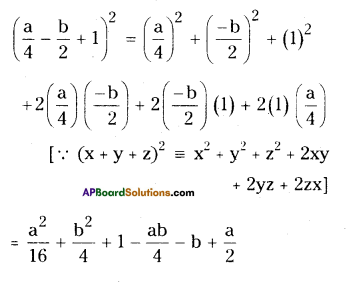v) (p + 1)3
Solution:
(p + 1)3
= (P)3 + 3 (p)2 (1) + 3 (p) (1)2 + (1)3
[ ∵ (x + y)3 = x3 + 3x2y + 3xy2 + y3]
= p3 + 3p2 + 3p + 1

vi) (𝑥−23𝑦)3
Solution: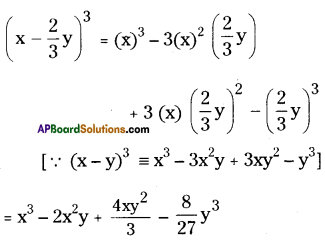Question 5.
Factorise
i) 25x2 + 16y2 + 4z2 – 40xy + 16yz – 20xz
Solution:
25x2 + 16y2 + 4z2 – 40xy + 16yz – 20xz
= (5x)2 + (- 4y)2 + (- 2z)2 + 2(5x) (- 4y) + 2 (- 4y) (- 2z) + 2 (- 2z) (5x)
= (5x – 4y – 2z)2 = (- 5x + 4y +, 2z)2

ii) 9a2 + 4b2 + 16c2 + 12ab – 16bc – 24ca
Solution:
9a2 + 4b2 + 16c2 + 12ab – 16bc -24ca
= (3a)2 + (2b)2 + (- 4c)2+ 2 (3a) (2b) + 2 (2b) (- 4c) + 2(- 4c) (3a)
= (3a + 2b – 4c)2

Question 6.
If a + b + c = 9 and ab + be + ca = 26, find a2 + b2 + c2.
Solution:
Given that a + b + c = 9
Squaring on both sides,
(a + b + c)2 = 92
⇒ a2+ b2 + c2+ 2 (ab + be + ca) = 81 ⇒ a2 + b2 + c2 = 81 – 2 (ab + be + ca)
(by problem)
= 81 – 2 x 26
= 81 – 52 = 29

Question 7.
Evaluate the following by using suit¬able identities. m EachgM)
i) (99)3
Solution:
(99)2 = (100 – 1)3
= 1003 – 3 (100)2 (1) + 3 (100) (1)2 – 13
[ ∵ (x – y)3 = x3 – 3x2y + 3xy2 + y3]
= 1000000 – 30000 + 300 – 1
= 970299

ii) (102)3
Solution:
(102)3 = (100 + 2)3
= 1003 + 3 (100)2 (2) + 3 (100) (2)2 + 23
[ ∵ (x + y)3 = x3 + 3x2y + 3xy2 + y3]
= 1000000 + 60000 + 1200 + 8
= 1061208

iii) (998)3
Solution:
(998)3 =(1000 – 2)3
[ ∵ (x – y)3 = x3 – 3x2y + 3xy2 – y3] = 10003– 3(1000)2(2) + 3(1000)(2)2– 23
= 1000000000 – 6000000 + 12000 – 8
= 994011992

iv) (1001)3
Solution:
(1001)3 = (1000 + 1)3 .
[ ∵ (x + y)3 = x3 + 3x2y + 3xy2 + y3] = 10003 + 3(1000)2(1) + 3(1000) (1)2 + 13
= 1000000000 + 3000000 + 3000 + 1
= 1003003001

Question 8.
Factorise each of the following.
i) 8a3 + b3 + 12a2 b + 6ab2
Solution:
8a3 + b3 + 12a2 b + 6ab2
= (2a)3 + (b)3 + 3 (2a)2 (b) + 3 (2a) (b)2
= (2a + b)3

ii) 8a3 – b3 – 12a2 b + 6ab2
Solution:
8a3 – b3 – 12a2 b + 6ab2
= (2a)3 – (b)3 – 3 (2a)2 (b) + 3 (2a) (b)2
= (2a – b)3

iii) 1 – 64a3 -12a + 48a2
Solution:
1 – 64a3 – 12a + 48a2
= (1)3 – (4a)3 – 3(1)2 (4a) + 3(1) (4a)2
= (1 – 4a)3

iv) 8𝑝3−125𝑝2+625𝑝−1125
Solution: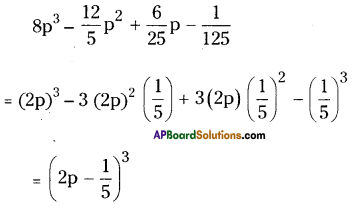Question 9.
Verify i) x3 + y3 = (x + y) (x2 – xy + y2);
ii) x3 – y3 = (x – y) (x2 + xy + y2)
Using some non-zero positive integers and check by actual multiplication. Can you
call these as identities ?
i) x3 + y3 = (x + y) (x2 – xy + y2)
Solution:
Given x3 + y3 = (x + y) (x2 – xy + y2)
L.H.S = x3 + y3
R.H.S = (x + y) (x2 – xy + y2)
= x (x2 – xy + y2) + y (x2 – xy + y2)
= x3 -x2y + xy2 + x2y – xy2 + y3
= x3 + y3
= L.H.S
∴ L.H.S = R.H.S

Take x = 3, y = 2
L.H.S = 33 + 23 = 27 + 8 = 35
R.H.S = (3 + 2) (32 – 3 x 2 + 22)
= 5 x (9 – 6 + 4)
= 5 x 7 = 35
∴ L.H.S = R.H.S

ii) x3 – y3 = (x – y) (x2 + xy + y2)
Solution:
Given that x3 – y3 = (x – y) (x2 + xy + y2)
L.H.S = x3 – y3
R.H.S = (x – y) (x2 + xy + y2)
= x (x2 + xy + y2) – y (x2 + xy + y2)
= x3 + x2y + xy2 – x2y – xy2 – y3
= x3 – y3= L.H.S

L.H.S = 33 – 23 = 27 – 8 = 19
R.H.S = (3 – 2) (32 + 3 x 2 + 22)
= 1 x (9 + 6 + 4)
= 1 x 19 = 19
∴ L.H.S = R.H.S
We can call the above two expressions as identities

Question 10.
Factorise by using the above results (identities).
i) 27a3 + 64b3
Solution:
27a3+ 64b3 = (3a)3 + (4b)3
= (3a + 4b) {(3a)2 – (3a) (4b) + (4b)2}
= (3a + 4b) (9a2 – 12ab + 16b2)

ii) 343y3 – 1000
Solution:
343y3 – 1000 = (7y)3 – (10)3
= (7y – 10) [(7y)2 + (7y) (10) + (10)2]
= (7y – 10) (49y2 + 70y + 100)

Question 11.
Factorise 27x3 + y3 + z3 – 9xyz using identity.
Solution:
Given 27x3 + y3 + z3 – 9xyz
= (3x)3 + (y)3 + (z)3 – 3 (3x) (y) (z)
= (3x + y + z)
[(3x)2 + y2 + z2 – (3x) (y) – (y) (z) – (z) (3x)]
[ ∵ (x3 + y3 + z3 – 3xyz = (x + y + z) (x2 + y2 + z2– xy – yz – zx)
= (3x + y + z) (9x2 + y2 + z2 – 3xy – yz – 3xz)

Question 12.
Verify that x3+ y3 + z3 – 3xyz = 1/2 (x + y + z) [(x – y)2 + (y – z)2 + (z – x)2 ]
(OR)
Verify that
p3 + q3 + r3 – 3pqr = 1/2 (p + q + r)
[(p – q)2 + (q – r)2 + (r – p)2]
Solution:
Given x3+ y3 + z3 – 3xyz = 1/2 (x + y + z) [(x – y)2 + (y – z)2 + (z – x)2 ]
R-H.S = 1/2 (x + y + z) [(x – y)2 + (y – z)2+ (z – x)2]
= 1/2 (x + y + z) [x2 + y2 – 2xy + y2 + z2 – 2yz + z2 + x2 – 2xz]
= 1/2 (x + y + z) [2x2 + 2y2 + 2z2 – 2xy – 2yz – 2zx]
= 1/2 (x + y + z) (2) [x2 + y2 + z2 – xy – yz – zx]
= (x + y + z) (x2 + y2 + z2 – xy – yz – zx)
= L.H.S
Hence proved.

Question 13.
If x + y + z = 0, show that x3 + y3 + z3 = 3xyz
Solution:
Given x + y + z = 0
To prove x3 + y3 + z3 = 3xyz
We have an identity
(x + y + z) (x2 + y2 + z2 – xy – yz – zx)
= x3 + y3 + z3 – 3xyz
Substituting x + y + z = 0in the above equation, we get
0 x (x2 + y2 + z2 -xy-yz-zx)
= x3 + y3 + z3 – 3xyz
⇒ x3 + y3 + z3 – 3xyz = 0
⇒ x3 + y3 + z3 = 3xyz

Question 14.
Without actual calculating the cubes, find the value of each of the following.
i) (- 10)3 + 73 + 33
Solution:
Given (-10)3 + 73 + 33
Sum of the bases = -10 + 7 + 3 = = 0
∴ (- 10)3 + 73 + 33
= 3 (- 10) x (7) x 3
= -630
[ ∵ x + y + z = 0 then x3 + y3 + z3 = 3xyz]

ii) (28)3 + (- 15)3 + (- 13)3
Solution:
Given (28)3 + (- 15)3+ (- 13)3
Sum of the bases = 28 + (- 15) + (- 13) = 0
∴ (28)3 + (- 15)3 + (- 13)3
= 3 x 28 x (- 15) x (- 13)
= 16380

iii) (12)3+(13)3−(56)3 read it as (12)3+(13)3+(−56)3
Solution: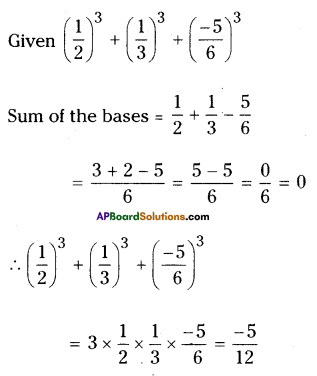iv) (0.2)3 – (0.3)3 + (0.1)3
Solution:
Given that (0.2)3 – (0.3)3 + (0.1)3
= (0.2)3 + (- 0.3)3 + (0.1)3
Sum of the bases = 0.2 – 0.3 + 0.1 = 0
∴ (0.2)3 + (-0.3)3 + (0.1)3
= 3 x (0.2) (- 0.3) (0.1)
= -0.018

Question 15.
Give possible expressions for the length and breadth of the rectangle whose area is given by
i) 4a2 + 4a – 3
Given that area = 4a2 + 4a – 3
= 4a2 + 6a – 2a – 3
= 2a (2a + 3) – 1 (2a + 3)
= (2a – 1) (2a + 3)
∴ Length = (2a + 3); breadth = (2a – 1).

ii) 25a– 35a + 12
Solution:
Given that area = 25a2 – 35a +12
= 25a2 – 20a – 15a + 12
= 5a (5a – 4) – 3 (5a – 4)
= (5a – 4) (5a – 3)
∴ (5a – 4) (5a – 3) are the length and breadth.

Question 16.
What are the possible polynomial expressions for the dimensions of the cuboids whose volumes are given below ?
i) 3x3 – 12x
Solution:
Volume = 3x– 12x
= 3x (x2 – 4)
= 3x (x + 2) (x – 2) are the dimensions.

ii) 12y2 + 8y – 20
Solution:
Given that volume = 12y2 + 8y – 20
= 4 (3y2 + 2y – 5)
= 4 [3y2 + 5y – 3y – 5]
= 4 [y (3y + 5) – 1 (3y + 5)]
= 4 (3y + 5) (y – 1)
Hence 4, (3y + 5) and (y – 1) are the dimensions.

Question 17.
Show that if 2 (a2 + b2 ) = (a + b)2 then a = b
Solution:
Given that 2 (a2 + b2 ) = (a + b)2
To prove a = b
As 2 (a2 + b2 ) = (a + b)2
We have
2a2 + 2b2 = a2 + 2ab + b2
2a2 – a2 + 2b2 – b2 = 2ab
a2 + b2 = 2ab
This is possible only when a = b
∴ a = b

## Andhra Pradesh Board Class 9th Maths Chapter 2 Polynomials and Factorisation Ex 2.5 Textbooks for Exam Preparations

Andhra Pradesh Board Class 9th Maths Chapter 2 Polynomials and Factorisation Ex 2.5 Textbook Solutions can be of great help in your Andhra Pradesh Board Class 9th Maths Chapter 2 Polynomials and Factorisation Ex 2.5 exam preparation. The AP Board STD 9th Maths Chapter 2 Polynomials and Factorisation Ex 2.5 Textbooks study material, used with the English medium textbooks, can help you complete the entire Class 9th Maths Chapter 2 Polynomials and Factorisation Ex 2.5 Books State Board syllabus with maximum efficiency.

## FAQs Regarding Andhra Pradesh Board Class 9th Maths Chapter 2 Polynomials and Factorisation Ex 2.5 Textbook Solutions

#### Can we get a Andhra Pradesh State Board Book PDF for all Classes?

Yes you can get Andhra Pradesh Board Text Book PDF for all classes using the links provided in the above article.

## Important Terms

Andhra Pradesh Board Class 9th Maths Chapter 2 Polynomials and Factorisation Ex 2.5, AP Board Class 9th Maths Chapter 2 Polynomials and Factorisation Ex 2.5 Textbooks, Andhra Pradesh State Board Class 9th Maths Chapter 2 Polynomials and Factorisation Ex 2.5, Andhra Pradesh State Board Class 9th Maths Chapter 2 Polynomials and Factorisation Ex 2.5 Textbook solutions, AP Board Class 9th Maths Chapter 2 Polynomials and Factorisation Ex 2.5 Textbooks Solutions, Andhra Pradesh Board STD 9th Maths Chapter 2 Polynomials and Factorisation Ex 2.5, AP Board STD 9th Maths Chapter 2 Polynomials and Factorisation Ex 2.5 Textbooks, Andhra Pradesh State Board STD 9th Maths Chapter 2 Polynomials and Factorisation Ex 2.5, Andhra Pradesh State Board STD 9th Maths Chapter 2 Polynomials and Factorisation Ex 2.5 Textbook solutions, AP Board STD 9th Maths Chapter 2 Polynomials and Factorisation Ex 2.5 Textbooks Solutions,
Share: